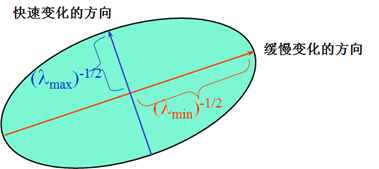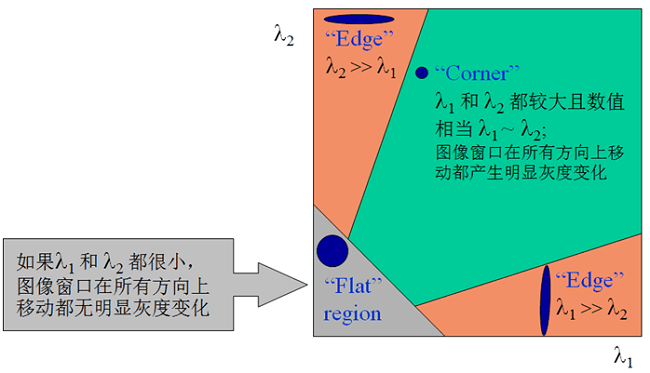# 基于Python的OpenCV图像处理16

## Jun 26,2017   6423 words   23 min

#### 二、图像中的角点

• 角点可以是两个边缘的交点
• 角点是邻域内具有两个主方向的特征点

#### 三、Moravec角点检测算子

• 如果窗口中的图像是平坦的，那么灰度变化不大
• 如果窗口中的图像是边缘，那么在沿边缘时变化不大，在垂直于边缘时变化较大
• 如果窗口中的图像是角点，那么沿任何方向，灰度变化都很大

$V(x,y)=\sum_{u,v}^{ }\left ( I_{x+u,y+v}-I_{x,y} \right )^{2}$

#### 四、Harris角点检测算子

##### 1.Harris算子原理推导

Harris角点检测算子其实是基于Moravec算子的改进，弥补了Moravec算子的不足，主要思想类似。 首先，将窗口平移(u,v)产生灰度变化的自相关函数如下：

$E(u,v)=\sum_{x,y}^{ }w(x,y)\left [ I(x+u,y+v)-I(x,y)\right ]^{2}$

$I(x+u,y+v)=I(x,y)+I_{x}u+I_{y}v+O(u^{2},v^{2})$

$E(u,v)=\sum_{x,y}^{ }w(x,y)\left [ I_{x}u+I_{y}v+O(u^{2},v^{2})\right ]^{2}$

$E(u,v)\approx \sum_{x,y}^{ }w(x,y)\left ( I_{x}u+I_{y}v\right )^{2}$

$(I_{x}u+I_{y}v)^{2} \\=u^{2}I_{x}^{2}+v^{2}I_{y}^{2}+2uvI_{x}I_{y} \\=\begin{bmatrix} u & v \end{bmatrix}\begin{bmatrix} I_{x}^{2} & I_{x}I_{y}\\ I_{y}I_{x} & I_{y}^{2} \end{bmatrix}\begin{bmatrix} u\\ v \end{bmatrix}$

$M=\sum_{x,y}^{ }w(x,y)\begin{bmatrix} I_{x}^{2} & I_{x}I_{y}\\ I_{y}I_{x} & I_{y}^{2} \end{bmatrix}$

$E(u,v)=\begin{bmatrix} u & v \end{bmatrix}M\begin{bmatrix} u\\ v \end{bmatrix}$

$\begin{bmatrix} u & v \end{bmatrix}M\begin{bmatrix} u\\ v \end{bmatrix}=1$椭圆函数特征值是由M决定，而M又是由图像不同位置的偏导数决定。特征值与图像中角点、边缘、平面之间的关系可分为三种情况：

• 图像中的直线:一个特征值大，另一个特征值小，λ1>λ2或λ2>λ1。自相关函数值在某一方向上大，在其它方向上小。
• 图像中的平面:两个特征值都小，且近似相等，自相关函数数值在各个方向上都小。
• 图像中的角点:两个特征值都大，且近似相等，自相关函数在所有方向都增大。$R=det(M)-k(trace(M))^{2}$

$trace(M) = \lambda _{1}+\lambda _{2}$ $det(M) = \lambda _{1}\lambda _{2}$

k为经验常数，一般取值为0.04 - 0.06。 增大k的值，将减小角点响应值R，降低角点检测的灵敏性，减少被检测角点的数量； 减小k值，将增大角点响应值R，增加角点检测的灵敏性，增加被检测角点的数量。

##### 2.Harris算子的性质

###### (2)Harris角点检测算子具有旋转不变性

Harris角点检测算子使用的是角点附近的区域灰度二阶矩矩阵。 而二阶矩矩阵可以表示成一个椭圆，椭圆的长短轴正是二阶矩矩阵特征值平方根的倒数。 当特征椭圆转动时，特征值并不发生变化，所以判断角点响应值R也不发生变化，由此说明Harris角点检测算子具有旋转不变性。

##### 3.OpenCV实现

###### (1)简单例子
# coding=utf-8
import cv2
import numpy as np
from matplotlib import pyplot as plt

gray = cv2.cvtColor(img, cv2.COLOR_BGR2GRAY)

# 由于Harris算子需要float32的输入图像，因此转换一下数据格式
gray = np.float32(gray)

# Harris算子
# 第一个参数是输入图像，数据类型为float32
# 第二个参数是角点检测中要考虑的领域大小
# 第三个参数是Sobel求导(Ix、Iy)时使用的窗口大小
# 第四个参数是Harris角点检测方程中的自由参数，0.04 - 0.06
dst = cv2.cornerHarris(gray, 2, 3, 0.04)

# 直接在原图上修改，也可以重新复制一份
# 0.01是人为设定的阈值
img[dst > 0.01 * dst.max()] = [0, 0, 255]

plt.imshow(dst, cmap='gray')
cv2.imshow("harris", img)
plt.show()
cv2.waitKey(0)


##### (2)复杂例子

# coding=utf-8
import cv2
import numpy as np

gray = cv2.cvtColor(img, cv2.COLOR_BGR2GRAY)

gray = np.float32(gray)

dst = cv2.cornerHarris(gray, 2, 3, 0.04)

ret, dst = cv2.threshold(dst, 0.01 * dst.max(), 255, cv2.THRESH_BINARY)

dst = np.uint8(dst)

# 找到重心
ret, labels, stats, centroids = cv2.connectedComponentsWithStats(dst)

# 定义结束条件
criteria = (cv2.TERM_CRITERIA_EPS + cv2.TERM_CRITERIA_MAX_ITER, 100, 0.001)

# 返回值是由角点坐标组成的数组而非图像
corners = cv2.cornerSubPix(gray, np.float32(centroids), (5, 5), (-1, -1), criteria)

# 将精细化前后的坐标放在一起
res = np.hstack((centroids, corners))

# np.int0用于省略小数点后的数字(截取)
# 由于绘制只能用int所以需要把亚像素转换成整像素
# 但是可以输出float类型的corner坐标，这是精确的
res = np.int0(res)

# 绘制角点，红色代表Harris角点，绿色代表修正后角点
img[res[:, 1], res[:, 0]] = [0, 0, 255]
img[res[:, 3], res[:, 2]] = [0, 255, 0]

# 输出精确坐标
print corners

cv2.imshow("harris", img)
cv2.waitKey(0)